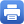Columbia Home
Algebraic Topology

This course is taken in sequence, part 1 in the fall, and part 2 in the spring.

Prerequisites:

• Basic notions of differentiable manifolds (see Chapter 0 and 1 of do Carmo’s Riemannian Geometry).
• Fundamental group, van Kampen’s theorem, covering spaces.
• Commutative algebra of PIDs (free modules, classification of finitely generated modules).

### FALL

Part I: Foundations of differential topology and homotopy theory

• Basic properties of higher homotopy groups
• Smooth manifolds, regular values and transversality
• Vector fields and the Poincaré-Hopf theorem
• Pontryagin construction
• Morse functions
• Topology of CW complexes

Part II: Homology and cohomology

• Homological algebra of chain complexes
• Singular and cellular homology
• The universal coefficient theorem
• Hurewicz theorem
• Cohomology and cup products
• Poincaré duality

### SPRING

Part I: Topology of fibrations

• Loopspaces
• Homology with local coefficients
• Obstruction theory
• Eilenberg MacLane spaces
• Filtered chain complexes and the associated spectral sequence
• The Serre spectral sequence of a fibration
• Multiplicative structures
• Applications to the computation of homotopy groups of spheres

Part II: Characteristic classes

• Classifying spaces of vector bundles
• Cohomology of Grassmannians
• Stiefel-Whitney, Chern, Pontryagin and Euler classes
• Thom spaces
• Computations of bordism groups
• Hirzebruch’s signature formula and Milnor’s exotic 7-spheres

Part III (time permitting): Further topics in Geometric TopologyPrint this page